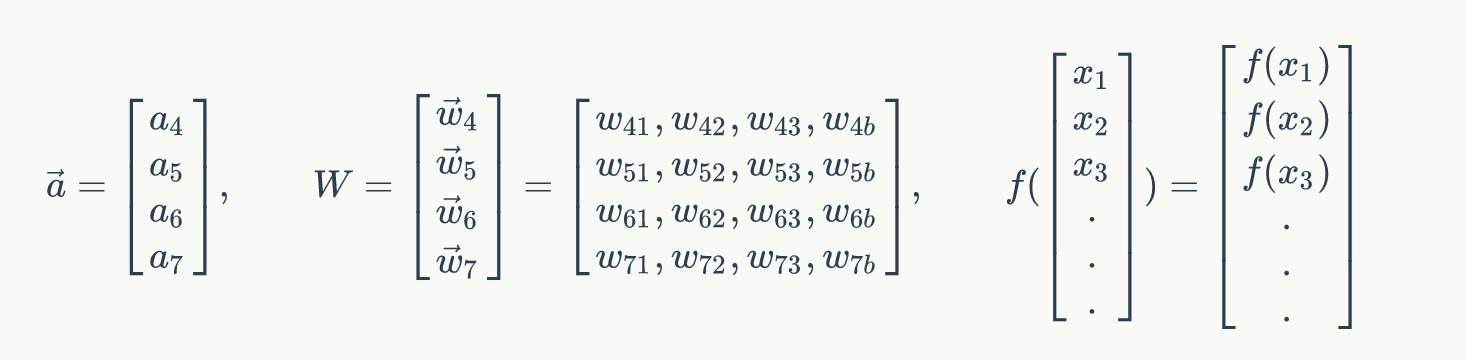# 神经网络基础与人工神经网络## 感知器• 输入权值，一个感知器可以有多个输入$x_1,x_2,x_3...x_n$,每个输入上有一个权值$w_i$
• 激活函数，感知器的激活函数有许多选择，以前用的是阶跃函数，$sigmoid\left(\frac{1}{1+e^{w*x}}\right)$,其中$z$为权重数据积之和
• 输出，$y{=}f\left({w*x}{+}{b}\right)$## 神经网络• 输入向量的维度和输入层神经元个数相同
• 第N层的神经元与第N-1层的所有神经元连接，也叫 全连接
• 上图网络中最左边的层叫做输入层，负责接收输入数据；最右边的层叫输出层，可以有多个输出层。我们可以从这层获取神经网络输出数据。输入层和输出层之间的层叫做隐藏层，因为它们对于外部来说是不可见的。
• 而且同一层的神经元之间没有连接
• 并且每个连接都有一个权值，${a_4}{=}sigmoid\left({W_{41}* x_1}{+}{W_{42}* x_2}{+}{W_{43}* x_3}{+}{W_{4b}}\right)$

${a_5}{=}sigmoid\left({W_{51}* x_1}{+}{W_{52}* x_2}{+}{W_{53}* x_3}{+}{W_{5b}}\right)$

${a_6}{=}sigmoid\left({W_{61}* x_1}{+}{W_{62}* x_2}{+}{W_{63}* x_3}{+}{W_{6b}}\right)$

${a_7}{=}sigmoid\left({W_{71}* x_1}{+}{W_{72}* x_2}{+}{W_{73}* x_3}{+}{W_{7b}}\right)$## 神经网络的训练

• 计算结果误差

• 通过梯度下降找到误差最小

• 更新权重以及偏置项

### 反向传播## tensorflow神经网络接口的实现

tf.train.GradientDescentOptimizer(0.5)


### 方法

init

__init__(
learning_rate,
use_locking=False,
)

• learning_rate tensor或者浮点值，用于学习速率

minimize

minimize(
loss,
global_step=None,
var_list=None,